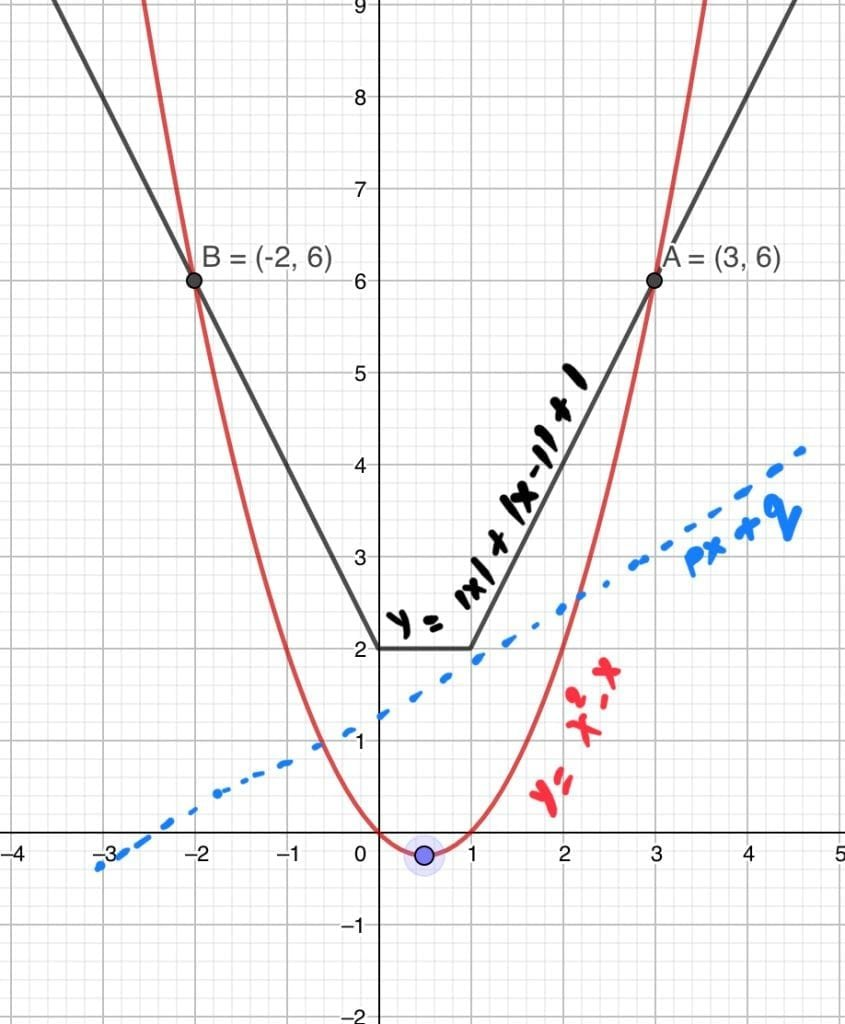How Cheenta works to ensure student success?
Explore the Back-Story

# Test of Mathematics Solution Subjective 50 -Dictionary RankingThis is a Test of Mathematics Solution Subjective 50 (from ISI Entrance). The book, Test of Mathematics at 10+2 Level is Published by East West Press. This problem book is indispensable for the preparation of I.S.I. B.Stat and B.Math Entrance.

Also visit: I.S.I. & C.M.I. Entrance Course of Cheenta

## Problem

All the permutation of the letters $a,b,c,d,e$ are written down and arranged in alphabetical order as in dictionary. Thus the arrangement $abcde$ is in first position and $abced$ is in second position. What is the position of the word $debac$?

## Solution:

According to the arrangement in a dictionary, number of words:

i) starting with $a$ = 4!

ii) starting with $b$ = 4!

iii) starting with $c$ = 4!

iv) starting with $da$ = 3!

v) starting with $db$ = 3!

vi) starting with $dc$ = 3!

vii) starting with $dea$ = 2!

viii) starting with $deba$ = 1!

The last case gives us the word itself. So the position of the word will be = $3*4! + 3*3! + 2! +1$ = $93$

$debac$ is in the $93^{rd}$ position.This is a Test of Mathematics Solution Subjective 50 (from ISI Entrance). The book, Test of Mathematics at 10+2 Level is Published by East West Press. This problem book is indispensable for the preparation of I.S.I. B.Stat and B.Math Entrance.

Also visit: I.S.I. & C.M.I. Entrance Course of Cheenta

## Problem

All the permutation of the letters $a,b,c,d,e$ are written down and arranged in alphabetical order as in dictionary. Thus the arrangement $abcde$ is in first position and $abced$ is in second position. What is the position of the word $debac$?

## Solution:

According to the arrangement in a dictionary, number of words:

i) starting with $a$ = 4!

ii) starting with $b$ = 4!

iii) starting with $c$ = 4!

iv) starting with $da$ = 3!

v) starting with $db$ = 3!

vi) starting with $dc$ = 3!

vii) starting with $dea$ = 2!

viii) starting with $deba$ = 1!

The last case gives us the word itself. So the position of the word will be = $3*4! + 3*3! + 2! +1$ = $93$

$debac$ is in the $93^{rd}$ position.

### Knowledge Partner